10 篇文章 29 订阅

1.插入排序

2.希尔排序

3.选择排序

4.堆排序

5.冒泡排序

6.快速排序

6.1 hoare版本

6.2挖坑法

6.3前后指针法

6.4快速排序优化

6.5快速排序非递归实现

7.归并排序

7.1递归实现

7.2非递归实现

8.计数排序(了解)

# 前言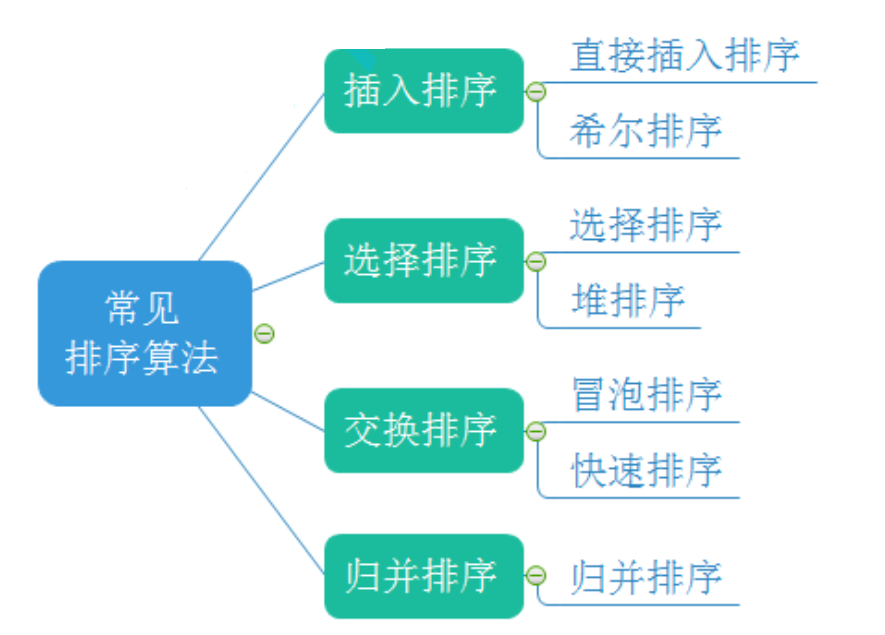// 排序实现的接口

// 插入排序
void InsertSort(int* a, int n);

// 希尔排序
void ShellSort(int* a, int n);

// 选择排序
void SelectSort(int* a, int n);

// 堆排序
void AdjustDwon(int* a, int n, int root);
void HeapSort(int* a, int n);

// 冒泡排序
void BubbleSort(int* a, int n);

// 快速排序递归实现

// 快速排序hoare版本
int PartSort1(int* a, int left, int right);

// 快速排序挖坑法
int PartSort2(int* a, int left, int right);

// 快速排序前后指针法
int PartSort3(int* a, int left, int right);
void QuickSort(int* a, int left, int right);

// 快速排序 非递归实现
void QuickSortNonR(int* a, int left, int right);

// 归并排序递归实现
void MergeSort(int* a, int n);

// 归并排序非递归实现
void MergeSortNonR(int* a, int n);

// 计数排序
void CountSort(int* a, int n);

# 常见排序算法的实现

## 1.插入排序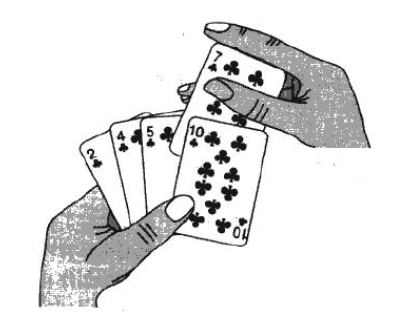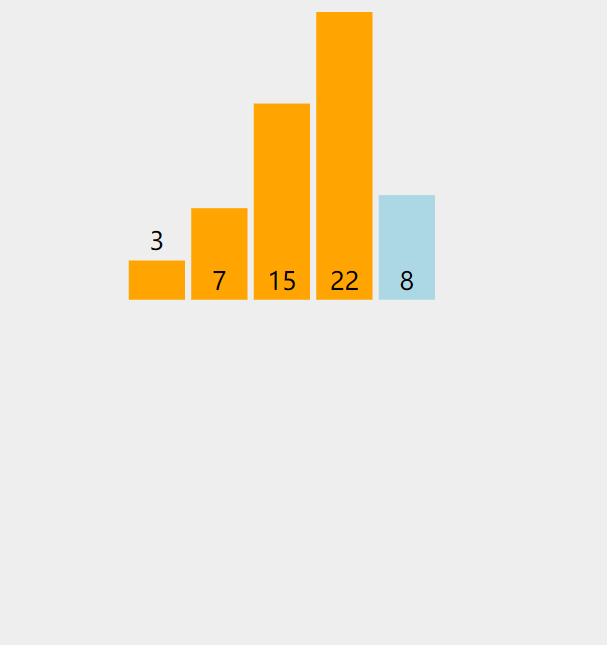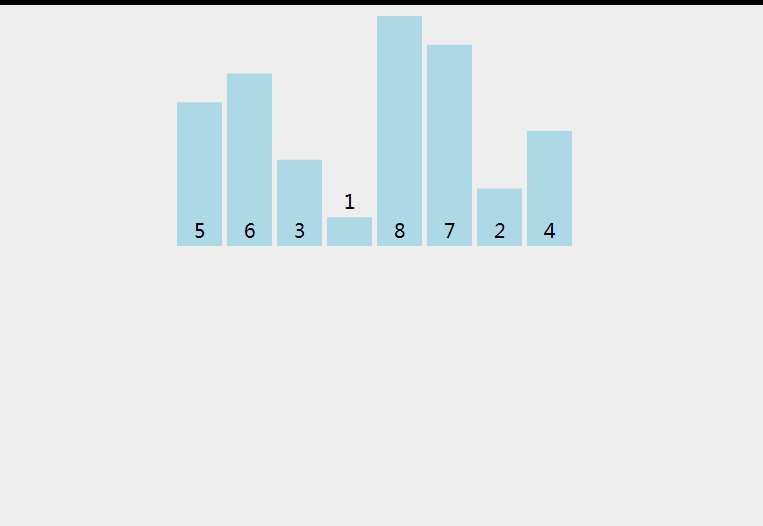// 插入排序
void InsertSort(int* a, int n)
{
assert(a);
int i = 0;
for (i = 0; i < n - 1; i++)//因为x元素位置是i的下一个位置，为防止x越界，需要使 i < n-1
{
int end = i;//已经有序的最后一个元素(一个元素不需要排序，所以默认从0开始)
int x = a[end + 1];//需要排序的元素

//单趟
while (end >= 0)
{

//若前一个数字大于x，则需将他向右移动
if (a[end] > x)
{
a[end + 1] = a[end];
//继续判断前面的元素
--end;
}

//前面元素小于x
else
{
break;
}
}

//将x插入正确位置(两种情况)
//1.前面的数字小于x
//2.前面的数字都大于x，x放在下标为0处
a[end + 1] = x;
}
}

1. 元素集合越接近有序，直接插入排序算法的时间效率越高
2. 时间复杂度：O(N^2)
3. 空间复杂度：O(1)，它是一种稳定的排序算法
4. 稳定性：稳定

## 2.希尔排序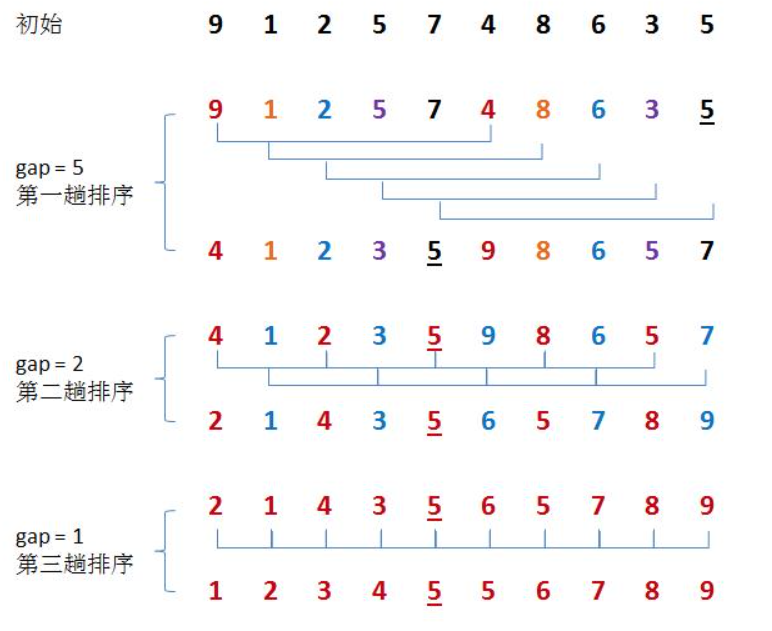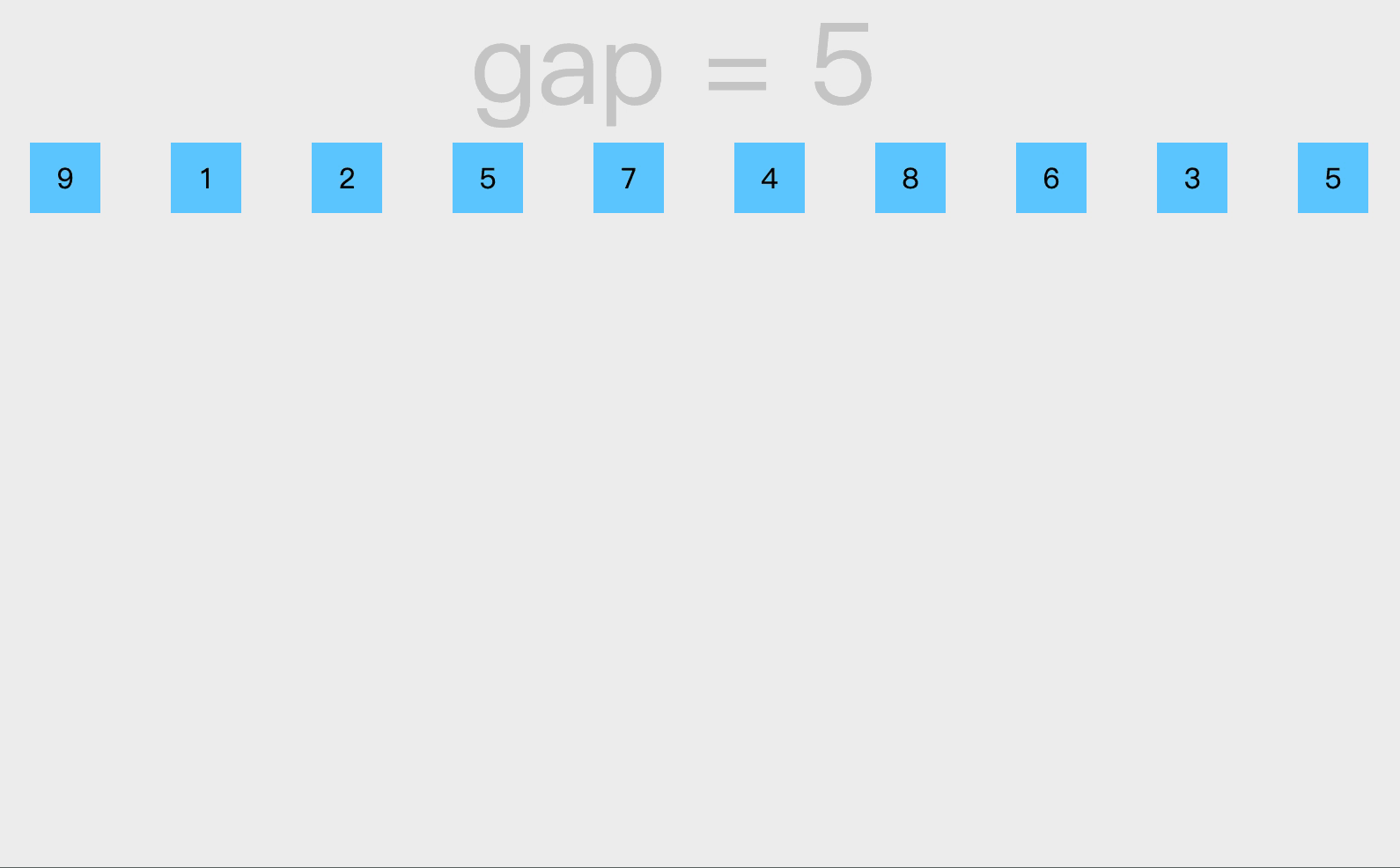// 希尔排序
void ShellSort(int* a, int n)
{
assert(a);
int gap = n;
while (gap > 1)
{
//gap /= 2;
gap = gap / 3 + 1;
for (int i = 0; i < n - gap; i++)
{
int end = i;
int x = a[end + gap];
while (end >= 0)
{
if (a[end] > x)
{
a[end + gap] = a[end];
end -= gap;
}
else
{
break;
}
}
a[end + gap] = x;
}
}
}

1. 希尔排序是对直接插入排序的优化。
2. 当gap > 1时都是预排序，目的是让数组更接近于有序。当gap == 1时，数组已经接近有序的了，这样就会很快。这样整体而言，可以达到优化的效果。
3. 希尔排序的时间复杂度不好计算，因为gap的取值方法很多，导致很难去计算，这里不深究。
4. 时间复杂度O(N^1.5)
5. 空间复杂度O(1)
6. 稳定性：不稳定。

## 3.选择排序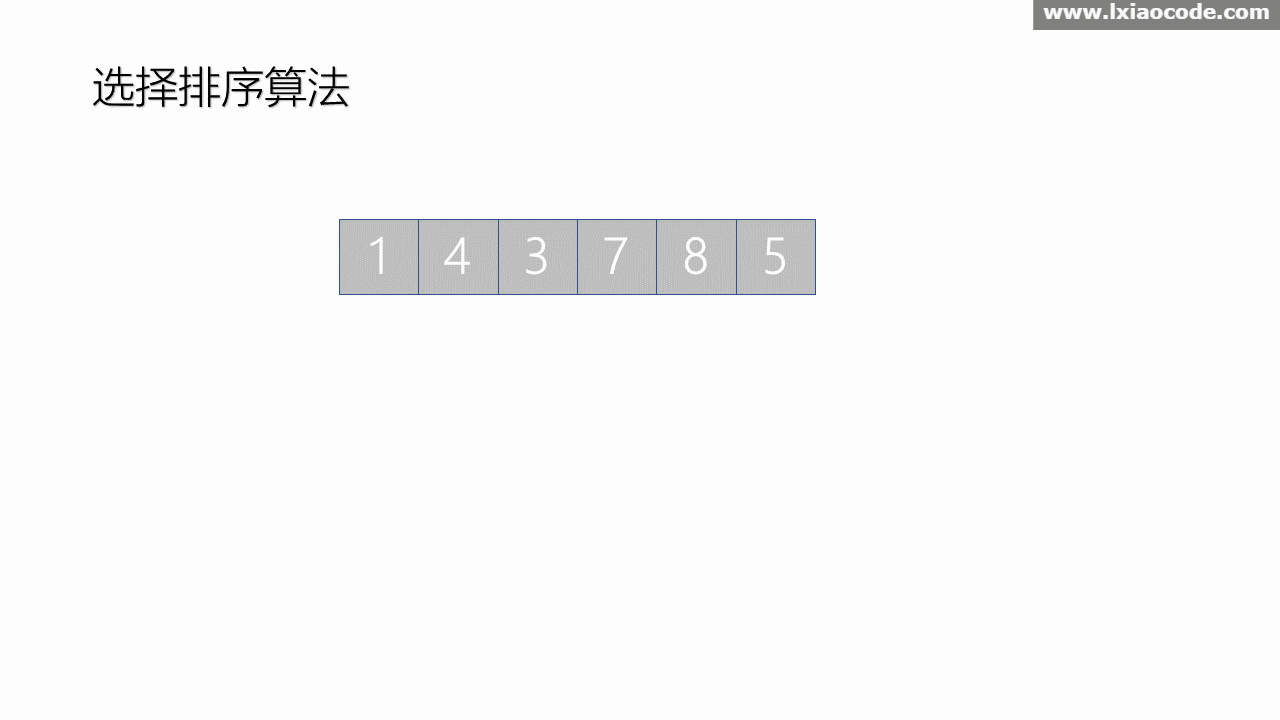// 选择排序
void SelectSort(int* a, int n)
{
assert(a);
int begin = 0;//保存数组的起始位置
int end = n - 1;//保存换数组的末尾位置

while (begin < end)
{
int maxi = begin;//保存最大元素下标
int mini = begin;//保存最小元素下标

//遍历数组寻找最小和最大元素
for (int i = begin; i <= end; i++)
{
if (a[i] < a[mini])
{
mini = i;
}
if (a[i] > a[maxi])
{
maxi = i;
}
}

//将最小元素交换到起始位置
Swap(a+begin, a+mini);

//判断最大值的位置是否在起始位置
if (maxi == begin)
{
maxi = mini;
}

//将最大元素交换到末尾位置
Swap(a+end, a+maxi);
//移动数组起始和末尾位置
begin++;
end--;
}
}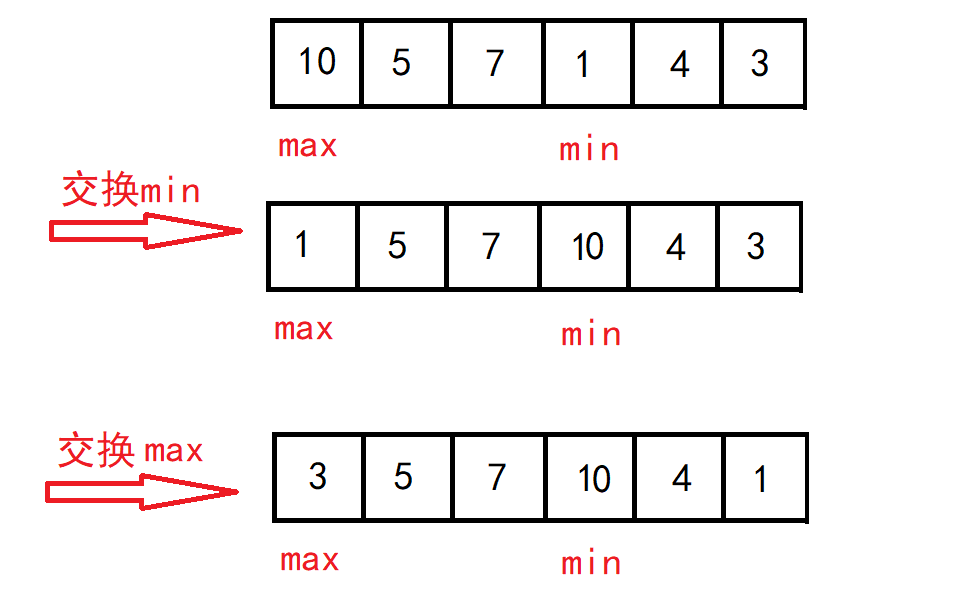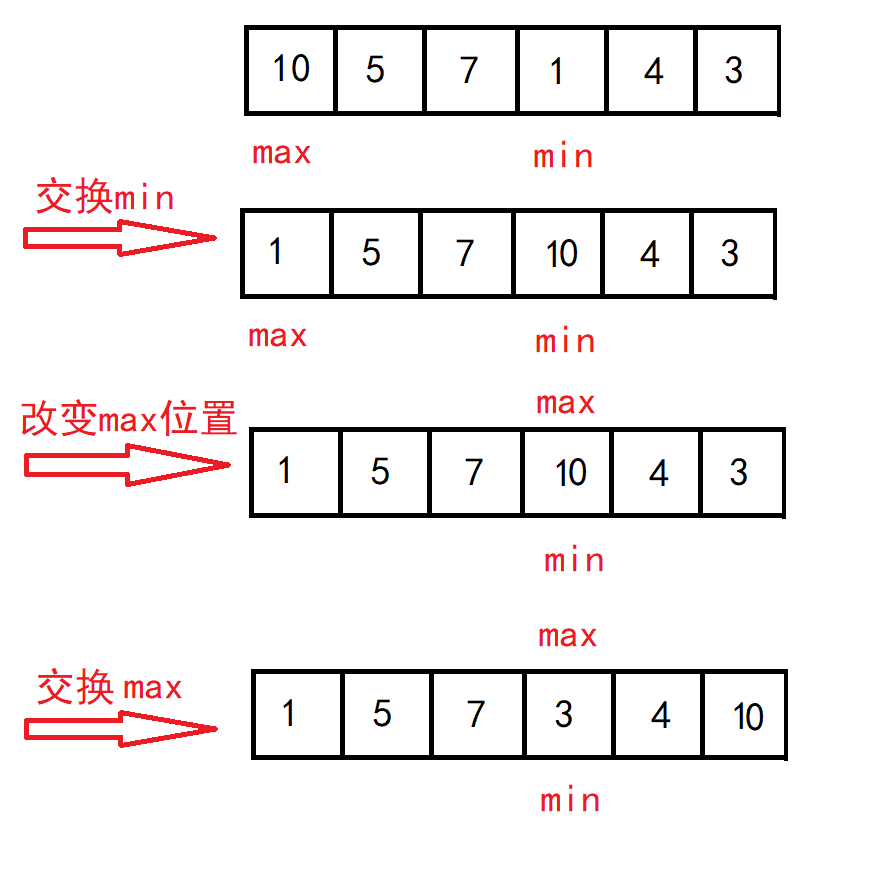直接选择排序的特性总结：

1.  直接选择排序思考非常好理解，但是效率不是很好(不论数组是否有序都会执行原步骤)。实际中很少使用
2.  时间复杂度：O(N^2)
3.  空间复杂度：O(1)
4.  稳定性：不稳定

## 4.堆排序

1. 建堆

• 升序：建大堆
• 降序：建小堆

2. 利用堆删除思想来进行排序

• 首先应该建一个大堆，不能直接使用堆来实现。可以将需要排序的数组看作是一个堆，但需要将数组结构变成堆。
• 我们可以从堆从下往上的第二行最右边开始依次向下调整直到调整到堆顶，这样就可以将数组调整成一个堆，且如果建立的是大堆，堆顶元素为最大值。
• 然后按照堆删的思想将堆顶和堆底的数据交换，但不同的是这里不删除最后一个元素。
• 这样最大元素就在最后一个位置，然后从堆顶向下调整到倒数第二个元素，这样次大的元素就在堆顶，重复上述步骤直到只剩堆顶时停止。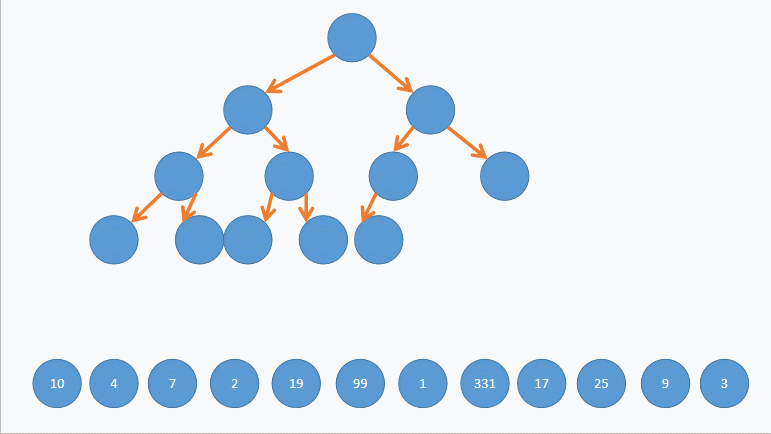// 堆排序
void AdjustDown(int* a, int n, int root)//向下调整
{
assert(a);
int parent = root;
int child = parent * 2 + 1;
while (child < n)
{
if (child + 1 < n && a[child + 1] > a[child])
{
child++;
}
if (a[child] > a[parent])
{
Swap(&a[child], &a[parent]);
parent = child;
child = parent * 2 + 1;
}
else
{
break;
}
}
}

void HeapSort(int* a, int n)
{
assert(a);

//建堆
for (int i = (n - 1 - 1) / 2; i >= 0; i--)
{
}

//交换
for (int i = n - 1; i > 0; i--)
{
Swap(&a[i], &a);
}
}

1. 堆排序使用堆来选数，效率就高了很多。
2. 时间复杂度：O(N*logN)
3. 空间复杂度：O(1)
4. 稳定性：不稳定

## 5.冒泡排序

(n-1趟是因为只剩两个元素时只需要一趟就可以完成)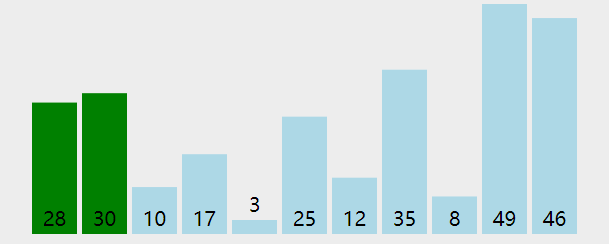// 冒泡排序
void BubbleSort(int* a, int n)
{
assert(a);
int i = 0;
int flag = 0;

//n-1趟排序
for (i = 0; i < n-1; i++)
{
int j = 0;

//一趟冒泡排序
for (j = 0; j < n - i - 1; j++)
{
if (a[j] > a[j+1])
{
Swap(&a[j], &a[j+1]);
flag = 1;
}
}

//若某一趟排序中没有元素交换则说明所有元素已经有序，不需要再排序
if (flag == 0)
{
break;
}
}
}

1. 冒泡排序是一种非常容易理解的排序
2. 时间复杂度：O(N^2)
3. 空间复杂度：O(1)
4. 稳定性：稳定

## 6.快速排序

### 6.1 hoare版本

1. 选定一个基准值，最好选定最左边或者最右边，选中间会给自己找麻烦。
2. 确定两个指针left 和right 分别从左边和右边向中间遍历数组。
3. 如果选最右边为基准值，那么left指针先走，如果遇到大于基准值的数就停下来。
4. 然后右边的指针再走，遇到小于基准值的数就停下来。
5. 交换left和right指针对应位置的值。
6. 重复以上步骤，直到left = right ，最后将基准值与left(right)位置的值交换。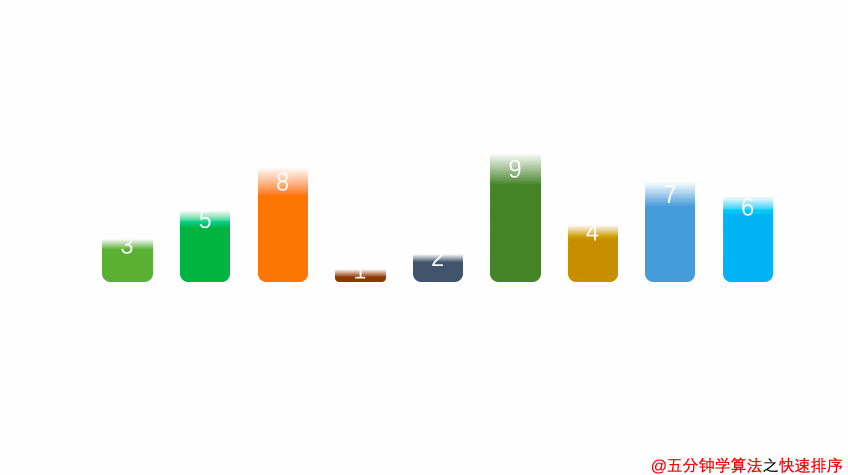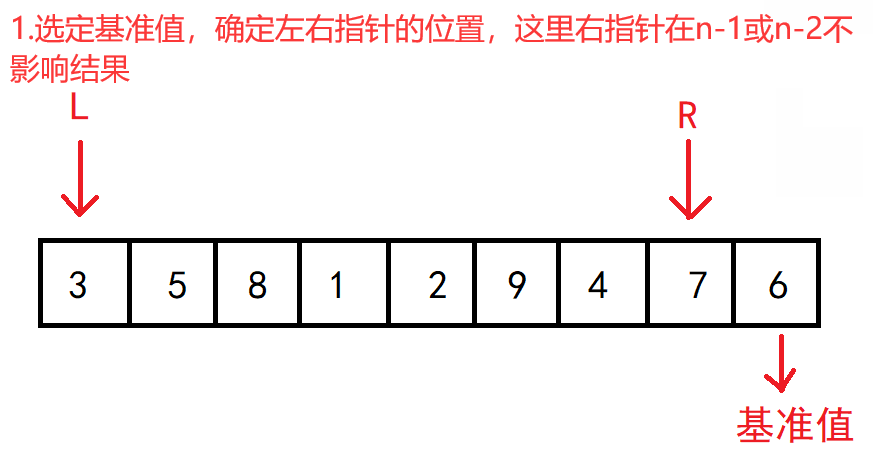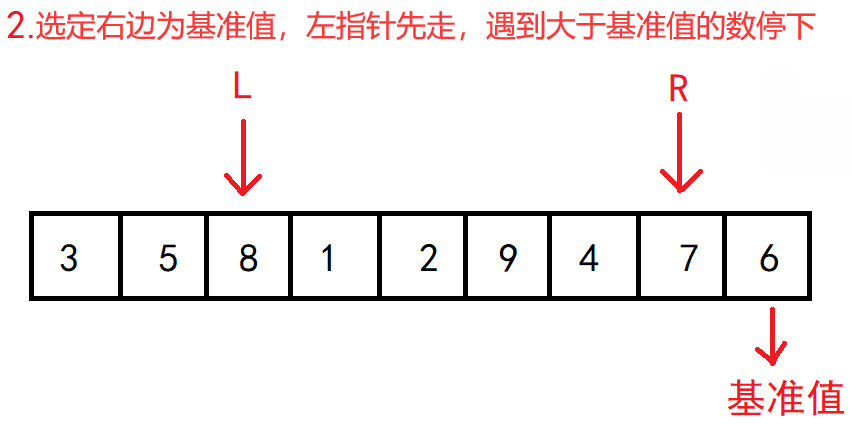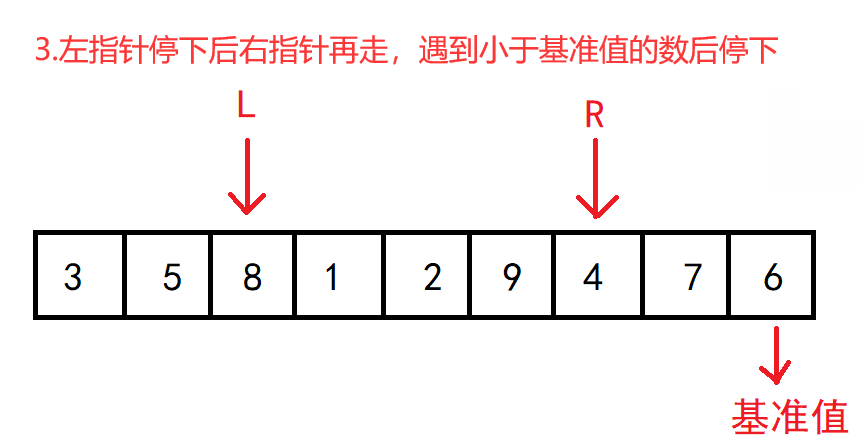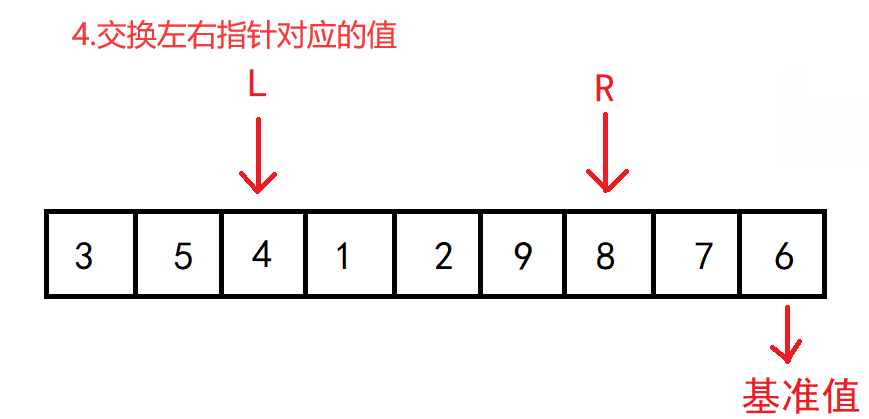继续按照上述步骤进行。。。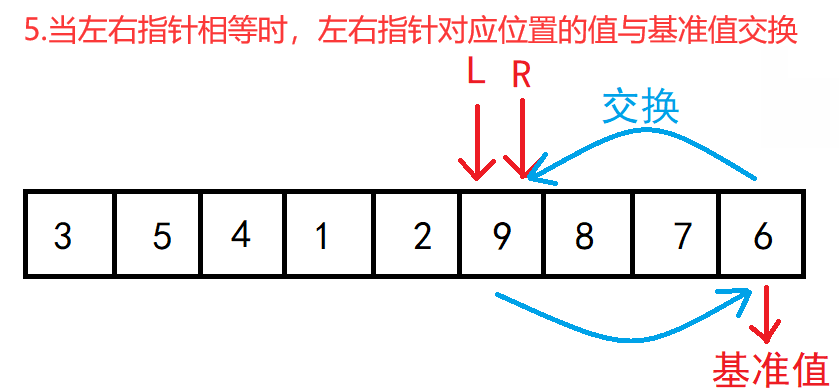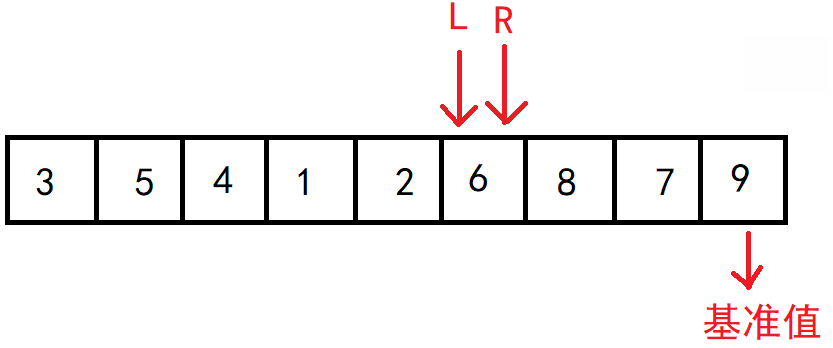// 快速排序hoare版本
int PartSort1(int* a, int left, int right)
{

int key = right;//选定基准值
while (left < right)
{
//选右边为基准值，左指针先走
while (left < right && a[left] <= a[key])
{
left++;
}

//右指针再走
while (left < right && a[right] >= a[key])
{
right--;
}

Swap(&a[left], &a[right]);
}
Swap(&a[left], &a[key]);
return left;
}

void QuickSort(int* a, int left, int right)
{
assert(a);
if (left >= right)
{
return;
}

int keyi = PartSort1(a, left, right);
QuickSort(a, left, keyi - 1);
QuickSort(a, keyi + 1, right);

}

### 6.2挖坑法

1. 先将选定的基准值(最左边)直接取出，然后留下一个坑，
2. 当右指针遇到小于基准值的数时，直接将该值放入坑中，而右指针指向的位置形成新的坑位，
3. 然后左指针遇到大于基准值的数时，将该值放入坑中，左指针指向的位置形成坑位，
4. 重复该步骤，直到左右指针相等。最后将基准值放入坑位之中。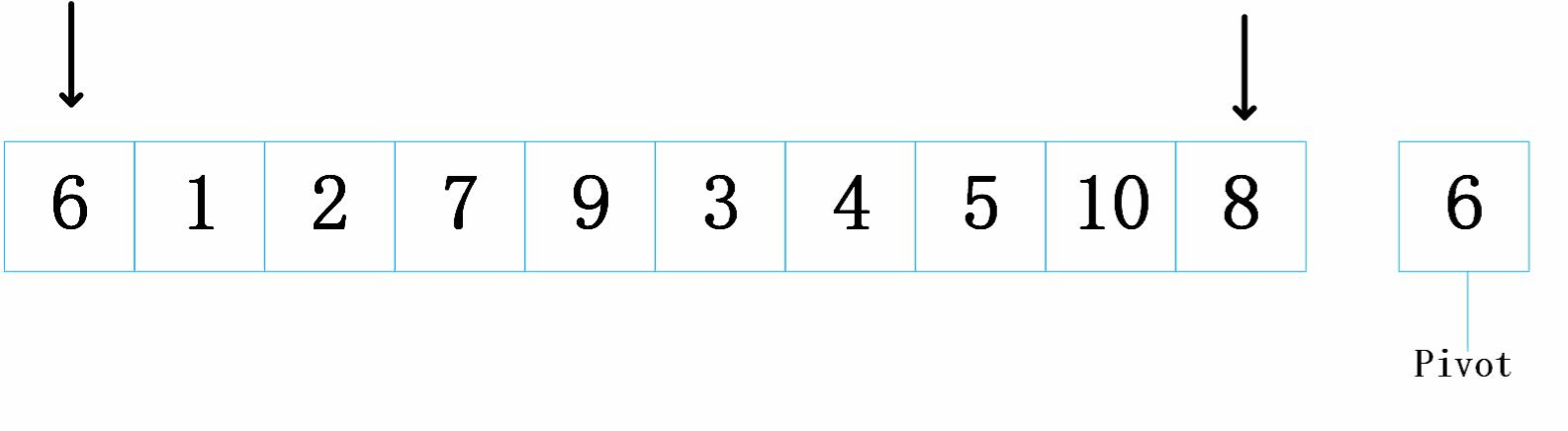// 快速排序挖坑法
int PartSort2(int* a, int left, int right)
{
int key = a[left];//取出基准值
int hole = left;//保存坑的位置
while (left < right)
{
while (left < right && a[right] >= key)
{
right--;
}
a[hole] = a[right];
hole = right;

while (left < right && a[left] <= key)
{
left++;
}
a[hole] = a[left];
hole = left;
}
a[hole] = key;
return hole;
}

void QuickSort(int* a, int left, int right)
{
assert(a);
if (left >= right)
{
return;
}

int keyi = PartSort2(a, left, right);
QuickSort(a, left, keyi - 1);
QuickSort(a, keyi + 1, right);

}

### 6.3前后指针法

1. 选定基准值，定义prev和cur指针(cur = prev + 1)
2. cur先走，遇到小于基准值的数停下，然后将prev向后移动一个位置
3. 将prev对应值与cur对应值交换
4. 重复上面的步骤，直到cur走出数组范围
5. 最后将基准值与prev对应位置交换
6. 递归排序以基准值为界限的左右区间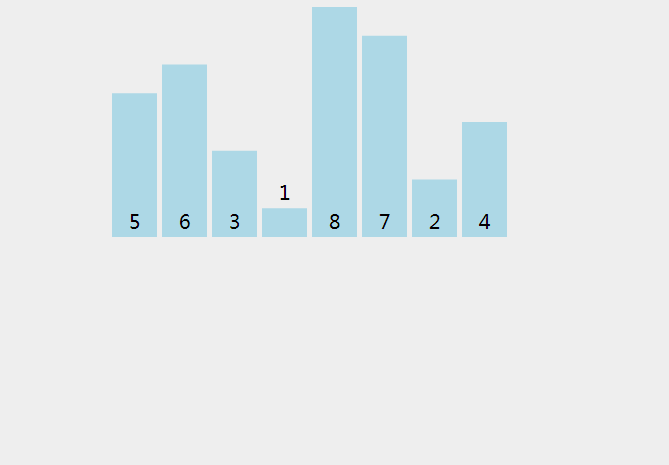单趟演示：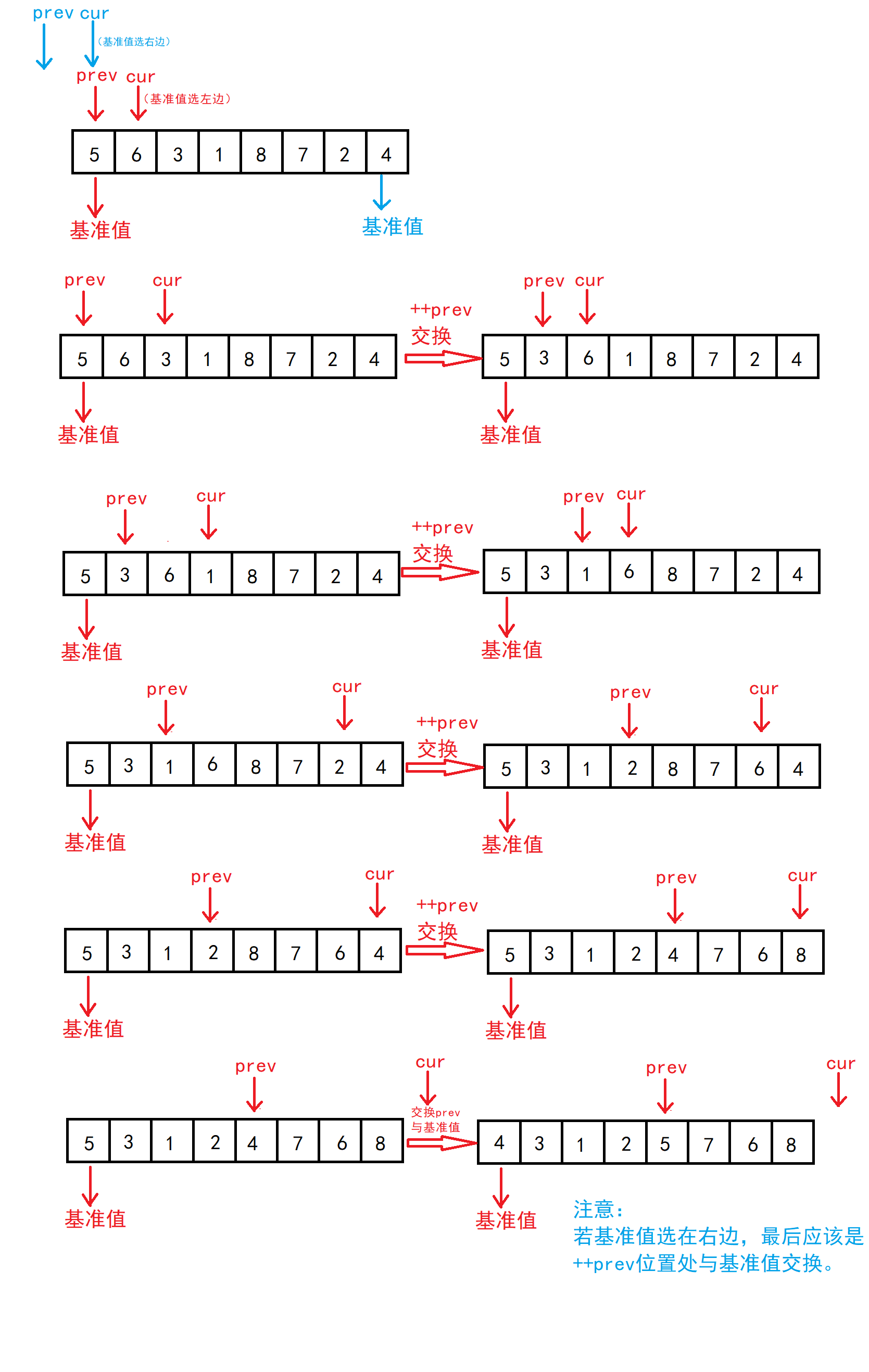// 快速排序前后指针法
int PartSort3(int* a, int left, int right)
{
//1.将基准值定在left
int keyi = left;
int prev = left;
int cur =  left + 1;
while (cur <= right)
{
if (a[cur] < a[keyi] && ++prev != cur)
{
Swap(&a[prev], &a[cur]);
}
cur++;

}
Swap(&a[prev], &a[keyi]);

//2.将基准值定在right
/*int keyi = right;
int prev = left - 1;
int cur = prev + 1;
while (cur <= right)
{
if (a[cur] < a[keyi] && ++prev != cur)
{
Swap(&a[cur], &a[prev]);
}
cur++;
}
Swap(&a[keyi], &a[++prev]);
return prev;*/
}

void QuickSort(int* a, int left, int right)
{
assert(a);
if (left >= right)
{
return;
}

int keyi = PartSort2(a, left, right);
QuickSort(a, left, keyi - 1);
QuickSort(a, keyi + 1, right);
}

### 6.4快速排序优化

1. 三数取中法选基准值
2. 递归到小的子区间时，可以考虑使用插入排序

1.即在在起始位置，中间位置，末尾位置中选出中间值，作为基准值。

//三数取中

int MidIndex(int* a, int left, int right)
{
int mid = (left + right) / 2;
//防止mid越界
//int mid = left+(right - left) / 2;

if (a[left] < a[right])
{
if (a[mid] < a[left])
{
return left;
}
else if (a[mid] > a[right])
{
return right;
}
else
{
return mid;
}
}
else
{
if (a[mid] > a[left])
{
return left;
}
else if (a[mid] < a[right])
{
return right;
}
else
{
return mid;
}
}
}

2.类似于二叉树，每个子树都会进行一次递归调用，越到下面递归调用会越多。为了减少递归调用，当到递归到下层时，我们可以使用其他的排序来替代。这里我们使用插入排序。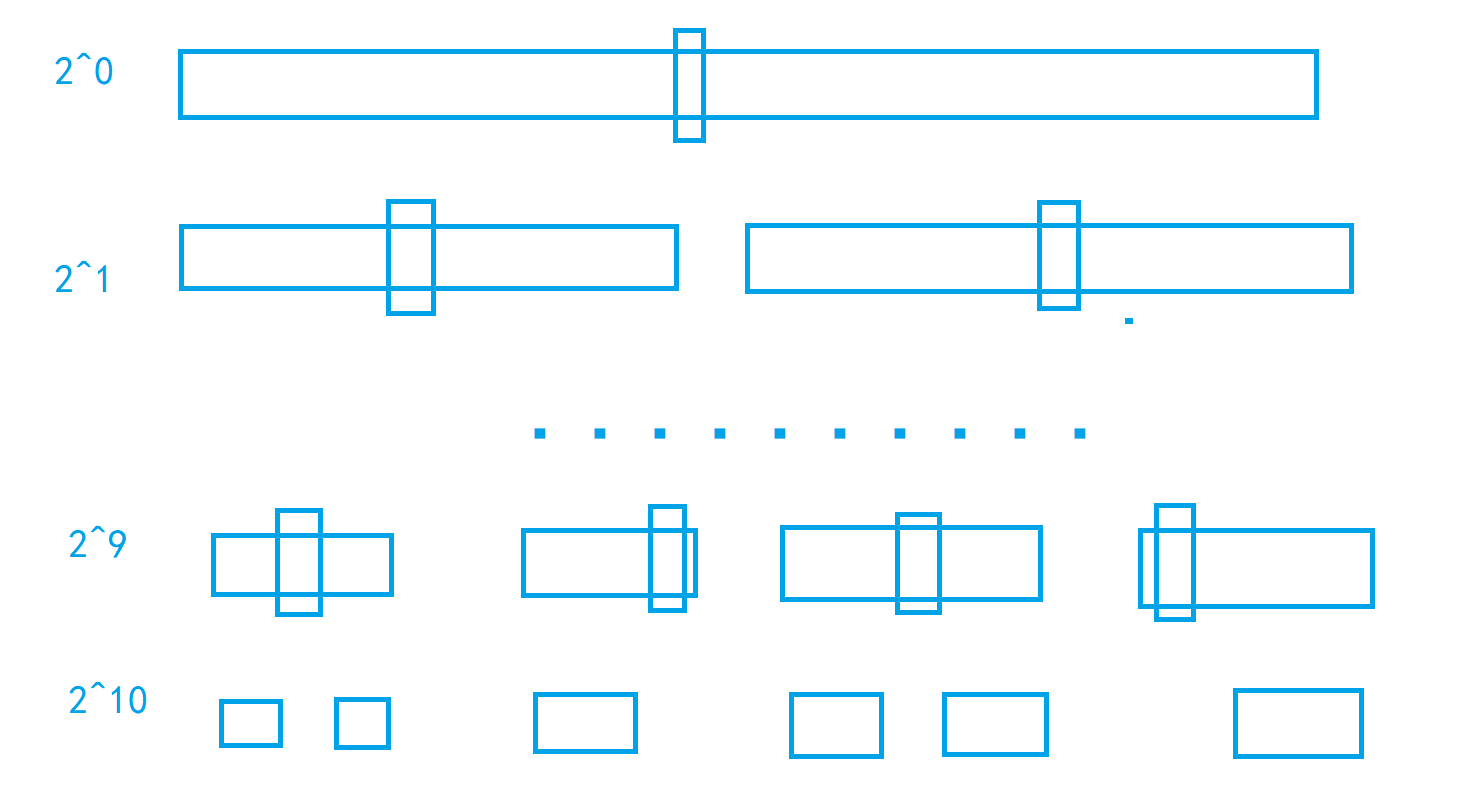// 快速排序前后指针法
int PartSort3(int* a, int left, int right)
{
int mid = MidIndex(a, left, right);
//将基准位置调整至最左边
Swap(&a[mid], &a[left]);
//1.将基准值定在left
int keyi = left;
int prev = left;
int cur =  left + 1;
while (cur <= right)
{
if (a[cur] < a[keyi] && ++prev != cur)
{
Swap(&a[prev], &a[cur]);
}
cur++;

}
Swap(&a[prev], &a[keyi]);

//2.将基准值定在right
/*int keyi = right;
int prev = left - 1;
int cur = prev + 1;
while (cur <= right)
{
if (a[cur] < a[keyi] && ++prev != cur)
{
Swap(&a[cur], &a[prev]);
}
cur++;
}
Swap(&a[keyi], &a[++prev]);
return prev;*/
}

void QuickSort(int* a, int left, int right)
{
assert(a);
if (left >= right)
{
return;
}

//小区间优化，，减少递归次数
if (right - left + 1 < 10)
{
InsertSort(a + left, right -left + 1);
}
else
{
int keyi = PartSort3(a, left, right);
QuickSort(a, left, keyi - 1);
QuickSort(a, keyi + 1, right);
}

}

### 6.5快速排序非递归实现

1. 将数组左右下标入栈，
2. 若栈不为空，两次取出栈顶元素，分别为闭区间的左右界限
3. 将区间中的元素按照前后指针法排序(其余两种也可)得到基准值的位置
4. 再以基准值为界限，若基准值左右区间中有元素，则将区间入栈
5. 重复上述步骤直到栈为空

// 快速排序 非递归实现
void QuickSortNonR(int* a, int left, int right)
{
//创建栈
Stack st;
StackInit(&st);

//原始数组区间入栈
StackPush(&st, right);
StackPush(&st, left);

//将栈中区间排序
while (!StackEmpty(&st))
{
//注意：如果right先入栈，栈顶为left
left = StackTop(&st);
StackPop(&st);
right = StackTop(&st);
StackPop(&st);

//得到基准值
int mid = PartSort3(a, left, right);

// 以基准值为分割点，形成左右两部分
if (right > mid+1)
{
StackPush(&st, right);
StackPush(&st, mid + 1);
}
if (left < mid - 1)
{
StackPush(&st, mid - 1);
StackPush(&st, left);
}
}
StackDestroy(&st);
}

1.  快速排序整体的综合性能和使用场景都是比较好的，所以才敢叫快速排序
2.  时间复杂度：O(N*logN)
3.  空间复杂度：O(logN)
4.  稳定性：不稳定

## 7.归并排序

### 7.1递归实现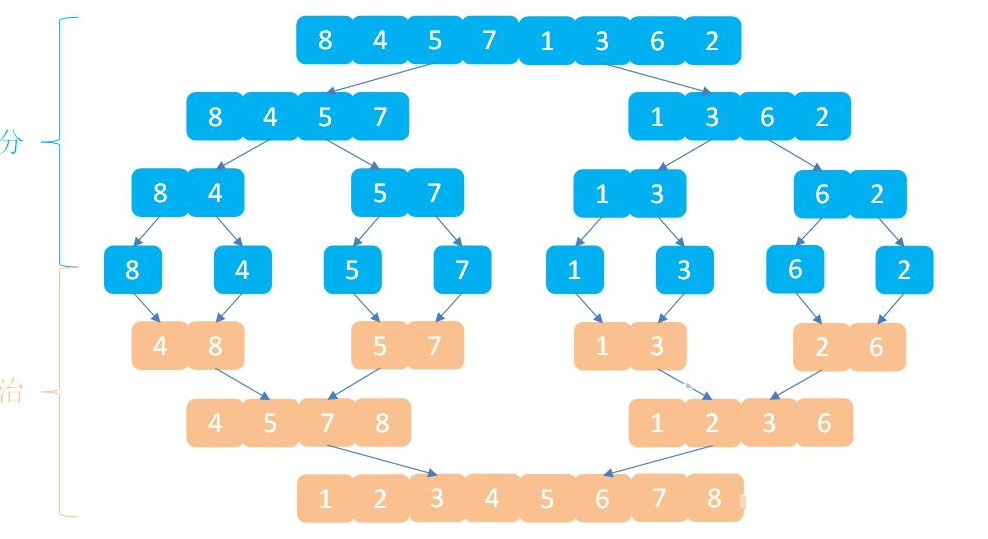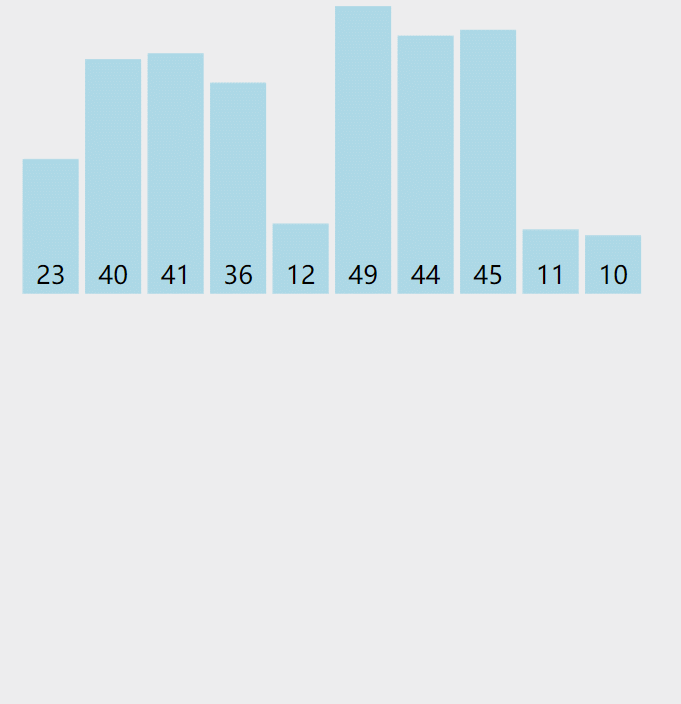void _MergeSort(int* a, int left, int right,int* tmp)
{
//区间中没有元素时不再合并
if (left >= right)
{
return;
}

//划分数组，每次一分为二
int mid = (left + right) / 2;
_MergeSort(a, left, mid,tmp);//划分左区间
_MergeSort(a, mid + 1, right,tmp);//划分右区间

//合并有序序列
int begin1 = left, end1 = mid;//有序序列1
int begin2 = mid + 1, end2 = right;//有序序列2
int i = left;
while (begin1 <= end1 && begin2 <= end2)//注意结束条件为一个序列为空时就停止
{
if (a[begin1] < a[begin2])
{
tmp[i++] = a[begin1++];
}
else
{
tmp[i++] = a[begin2++];
}
}

//两序列不可能同时为空，将剩余元素合并
while (begin1 <= end1)
{
tmp[i++] = a[begin1++];
}

while (begin2 <= end2)
{
tmp[i++] = a[begin2++];
}

//将合并后的序列拷贝到原数组中
//在这里拷贝的原因是 保证返回到上一层递归后两个子序列中的元素是有序的
int j = 0;
for (j = left; j <= right; j++)
{
a[j] = tmp[j];
}
}

// 归并排序递归实现
void MergeSort(int* a, int n)
{
assert(a);
//因为需要将两个有序序列合并，需借助额外数组
int* tmp = (int*)malloc(sizeof(int) * n);
if (tmp == NULL)
{
perror("malloc");
exit(-1);
}

_MergeSort(a, 0, n - 1,tmp);

free(tmp);
tmp = NULL;
}

### 7.2非递归实现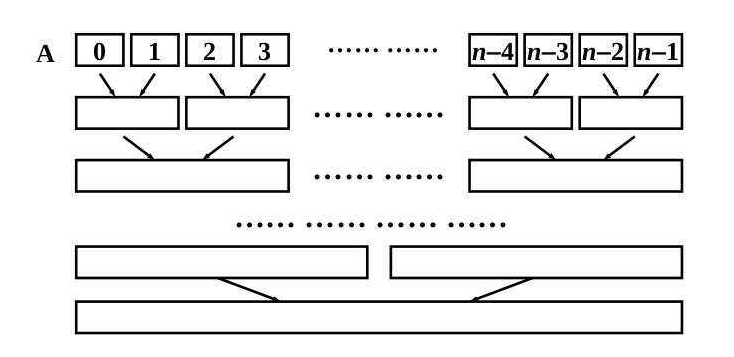// 归并排序非递归实现
void MergeSortNonR(int* a, int n)
{
assert(a);
int* tmp = (int*)malloc(sizeof(int) * n);
if (tmp == NULL)
{
perror("malloc");
exit(-1);
}

//初始化每组的元素个数为1
int gap = 1;
while (gap < n)//gap为n时就只有一个序列了，所以gap<n
{
//归并每两组归并一次
int i = 0;
for (i = 0; i < n; i+=2*gap)//两组中的元素个数为2*gap
{
//控制两组边界
int begin1 = i, end1 = i + gap - 1;
int begin2 = i + gap, end2 = i + 2 * gap - 1;

//当原数组中元素个数不是2^n时，最后两组组会出现元素不匹配的情况
//情况1：end1>=n或begin2>=n,即最后两组中只有一组有元素，则不需归并
if (end1 >= n || begin2 >= n)
{
break;
}
//情况2：end2>=n，即最后两组中，第二组元素个数小于第一组，则需要调整第二组边界
if (end2 >= n)
{
end2 = n - 1;
}

//归并
int j = i;
while (begin1 <= end1 && begin2 <= end2)
{
if (a[begin1] < a[begin2])
{
tmp[j++] = a[begin1++];
}
else
{
tmp[j++] = a[begin2++];
}
}

while (begin1 <= end1)
{
tmp[j++] = a[begin1++];
}

while (begin2 <= end2)
{
tmp[j++] = a[begin2++];
}

//将归并后的有序序列拷贝到原数组中
for (j = i; j <= end2; j++)
{
a[j] = tmp[j];
}
}
//每次循环每组元素个数增大2倍
gap *= 2;
}

free(tmp);
tmp = NULL;
}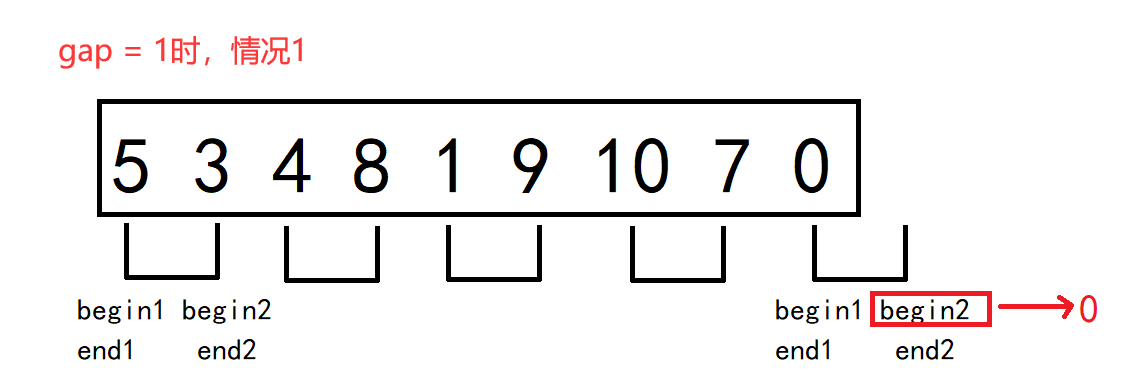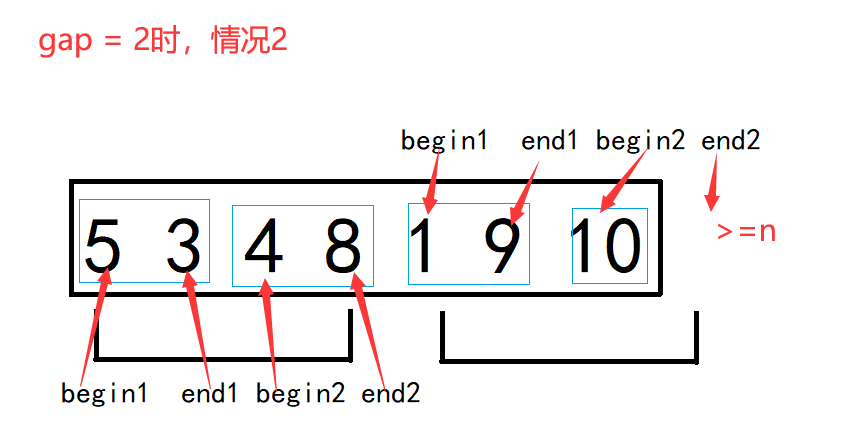1.  归并的缺点在于需要O(N)的空间复杂度，归并排序的思考更多的是解决在磁盘中的外排序问题。
2.  时间复杂度：O(N*logN)
3.  空间复杂度：O(N)
4.  稳定性：稳定

## 8.计数排序(了解)

1. 统计相同元素出现次数
2. 根据统计的结果将序列回收到原来的序列中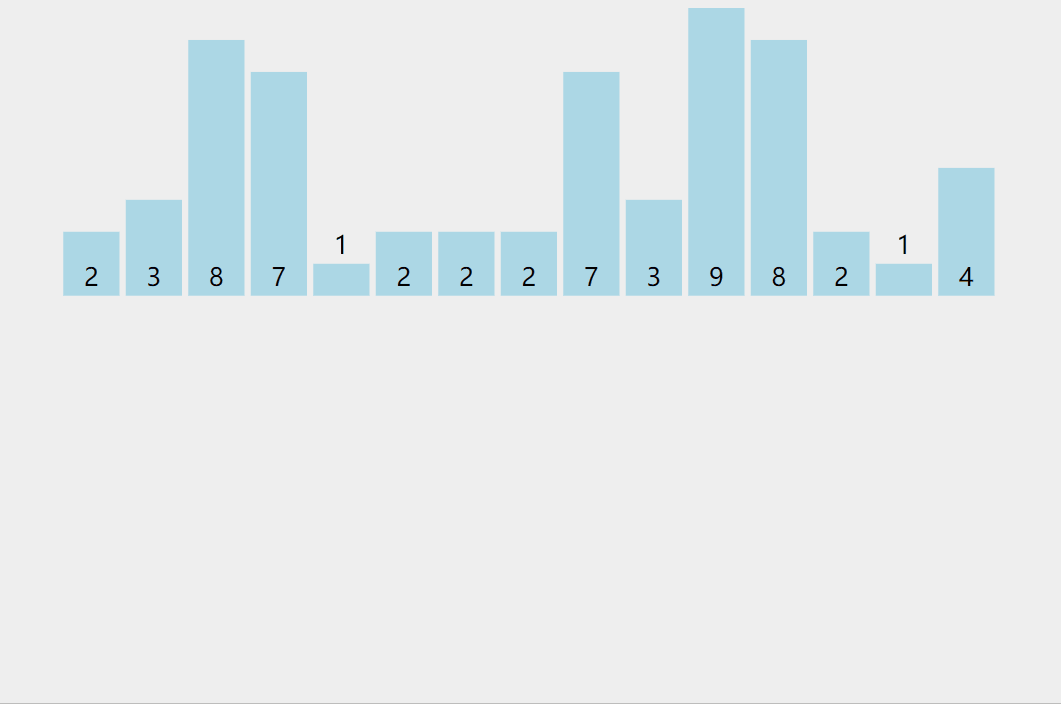代码如下：

// 计数排序
void CountSort(int* a, int n)
{
assert(a);
// 创建计数数组，数组大小为原数组中最大值-最小值+1
int max = a, min = a;
int i = 0;
for (i = 0; i < n; i++)
{
if (a[i] > max)
{
max = a[i];
}
if (a[i] < min)
{
min = a[i];
}
}
int range = max - min + 1;
int* count = (int*)malloc(sizeof(int) * range);
// 初始化计数数组为0
memset(count, 0, range * 4);

// 统计次数
for (i = 0; i < n; i++)
{
count[a[i] - min]++;
}
// 根据次数，进行排序
int j = 0;
for (i = 0; i < range; i++)
{
while (count[i]--)
{
a[j++] = i+min;
}
}
}

1.  计数排序在数据范围集中时，效率很高，但是适用范围及场景有限。
2.  时间复杂度：O(MAX(N,范围))
3.  空间复杂度：O(范围)
4. 稳定性：稳定

# 排序算法复杂度及稳定性分析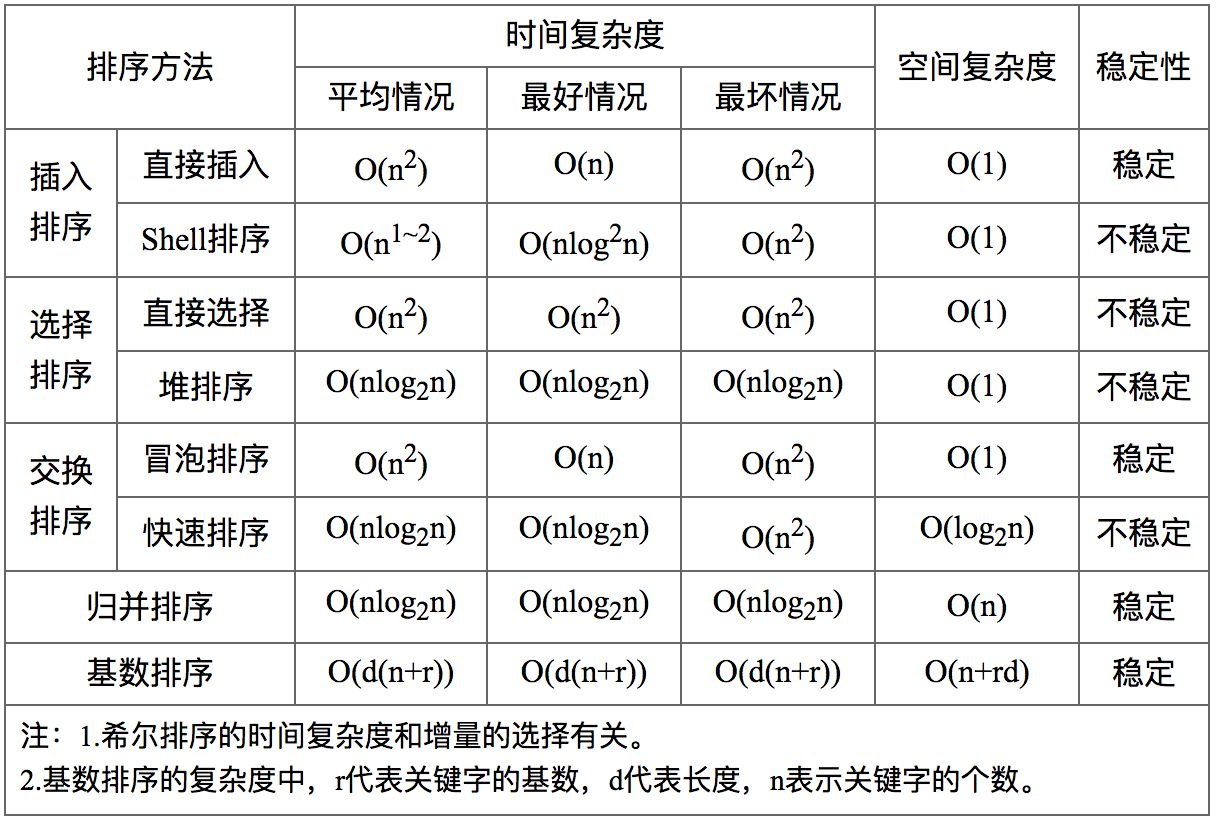void TestOP()
{
srand(time(0));
const int N = 100000;
int* a1 = (int*)malloc(sizeof(int) * N);
int* a2 = (int*)malloc(sizeof(int) * N);
int* a3 = (int*)malloc(sizeof(int) * N);
int* a4 = (int*)malloc(sizeof(int) * N);
int* a5 = (int*)malloc(sizeof(int) * N);
int* a6 = (int*)malloc(sizeof(int) * N);
for (int i = 0; i < N; ++i)
{
a1[i] = rand();
a2[i] = a1[i];
a3[i] = a1[i];
a4[i] = a1[i];
a5[i] = a1[i];
a6[i] = a1[i];
}
int begin1 = clock();
InsertSort(a1, N);
int end1 = clock();
int begin2 = clock();
ShellSort(a2, N);
int end2 = clock();
int begin3 = clock();
SelectSort(a3, N);
int end3 = clock();
int begin4 = clock();
HeapSort(a4, N);
int end4 = clock();
int begin5 = clock();
QuickSort(a5, 0, N - 1);
int end5 = clock();
int begin6 = clock();
MergeSort(a6, N);
int end6 = clock();
printf("InsertSort:%d\n", end1 - begin1);
printf("ShellSort:%d\n", end2 - begin2);
printf("SelectSort:%d\n", end3 - begin3);
printf("HeapSort:%d\n", end4 - begin4);
printf("QuickSort:%d\n", end5 - begin5);
printf("MergeSort:%d\n", end6 - begin6);
free(a1);
free(a2);
free(a3);
free(a4);
free(a5);
free(a6);
}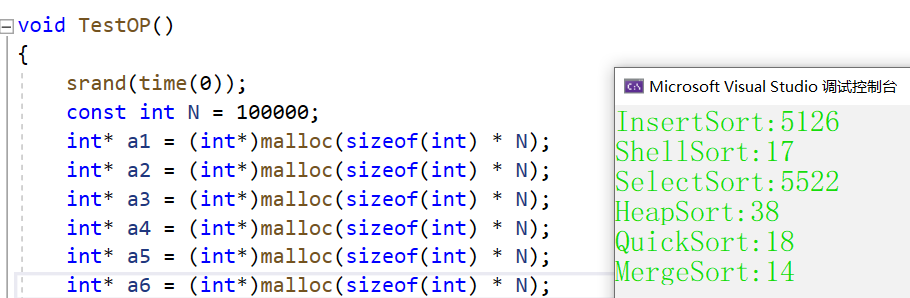08-295万+07-043406
03-312720
07-03729
02-1665
08-21656
03-011877
02-201万+
01-26
08-15376
12-06262
03-09625
10-02747
04-141万+
03-042万+
07-087123
08-11143点击重新获取扫码支付余额充值